Homework Help Question & Answers

# 7.30 Given the probability density function 20x3 (1- x) for 0< f(x) <1 and 0 elsewhere find the following: The cu...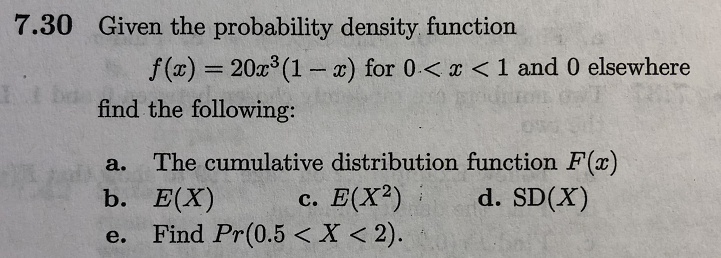7.30 Given the probability density function 20x3 (1- x) for 0

#### Homework Answers

Answer #1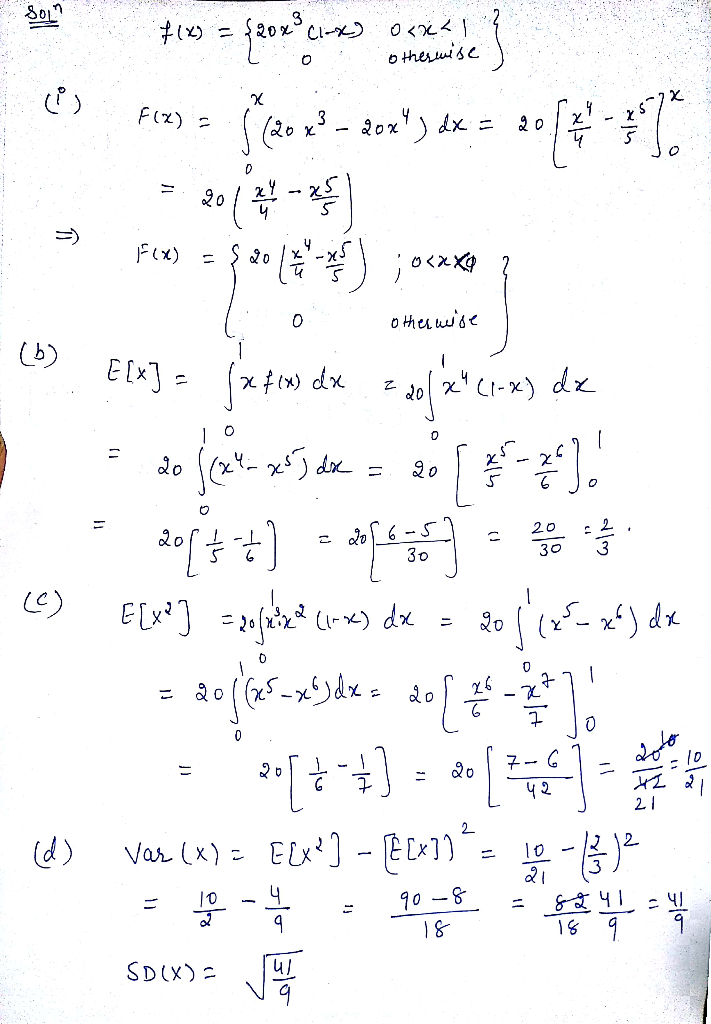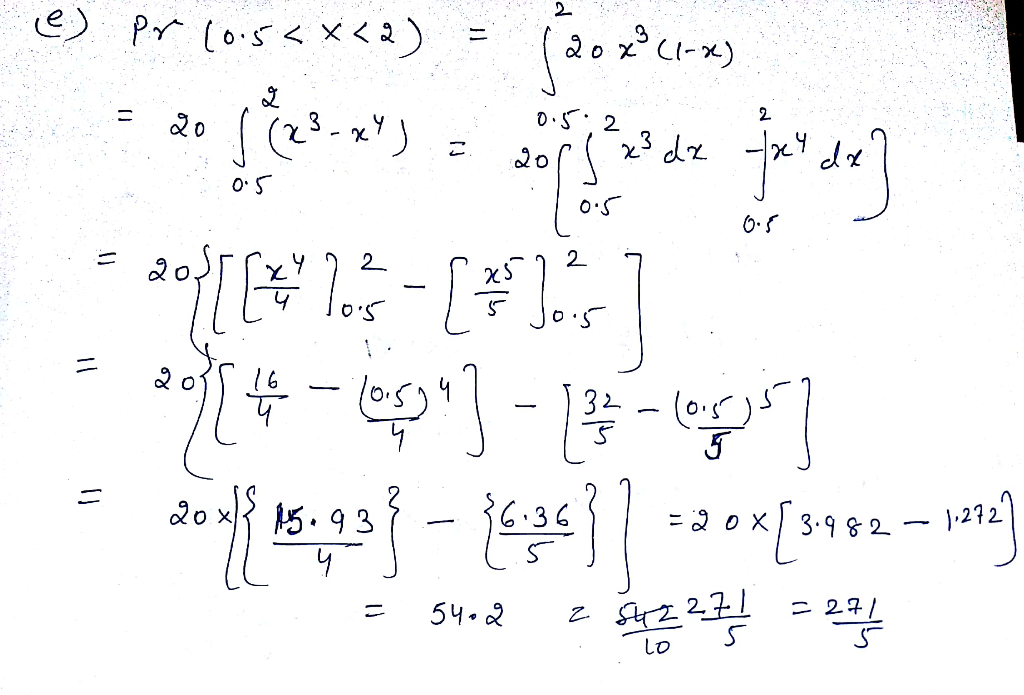Know the answer?
Your Answer:

#### Post as a guest

Your Name:

What's your source?

#### Earn Coin

Coins can be redeemed for fabulous gifts.

Not the answer you're looking for? Ask your own homework help question. Our experts will answer your question WITHIN MINUTES for Free.
Similar Homework Help Questions
• ### The joint probability density function of X and Yis defined by f(, )0 elsewhere What is Pr(X Y K ...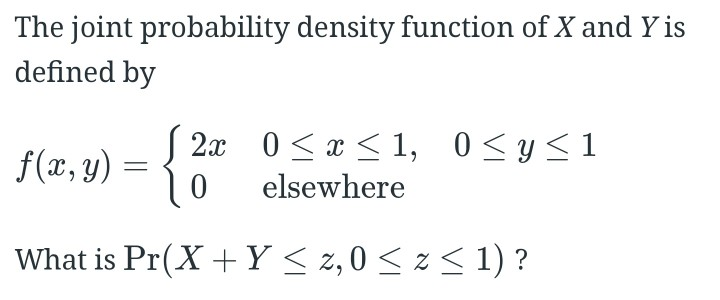The joint probability density function of X and Yis defined by f(, )0 elsewhere What is Pr(X Y K z,0 1)? The joint probability density function of X and Yis defined by f(, )0 elsewhere What is Pr(X Y K z,0 1)?

• ### The joint probability density function (pdf) of (X,Y ) is given by f(X,Y )(x,y) = 12/ 7 x(x + y), for 0 ≤ y ≤ 1, 0 ≤ x ≤ 1, 0, elsewhere. (a) Find the cumulative distribution function of (X,Y ). Make...

The joint probability density function (pdf) of (X,Y ) is given by f(X,Y )(x,y) = 12/ 7 x(x + y), for 0 ≤ y ≤ 1, 0 ≤ x ≤ 1, 0, elsewhere. (a) Find the cumulative distribution function of (X,Y ). Make sure you derive expressions for the cdf in the regions • x < 0 or y < 0; • 0 ≤ x ≤ 1, 0 ≤ y ≤ 1; • x > 1, 0 ≤ y ≤...

• ### Let X be a random variable with probability density function (pdf) given by fx(r0)o elsewhere where θ 0 is an unknown parameter. (a) Find the cumulative distribution function (cdf) for the random var...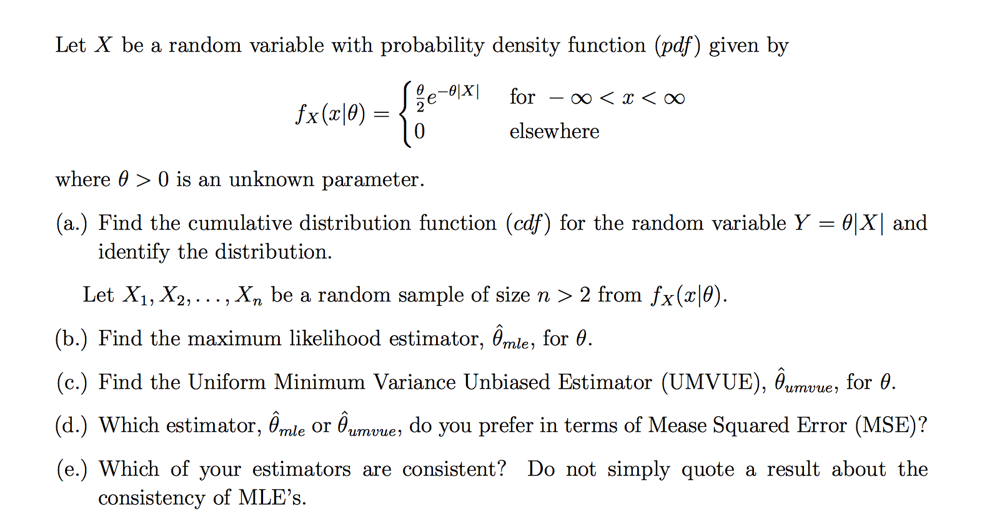Let X be a random variable with probability density function (pdf) given by fx(r0)o elsewhere where θ 0 is an unknown parameter. (a) Find the cumulative distribution function (cdf) for the random variable Y = θ and identify the distribution. Let X1,X2, . . . , Xn be a random sample of size n 〉 2 from fx (x10). (b) Find the maximum likelihood estimator, Ỗmle, for θ (c.) Find the Uniform Minimum Variance Unbiased Estimator (UMVUE), Bumvue, for 0...

• ### 2. The probability density function of X is given by 10 0,x < 10 a) Find...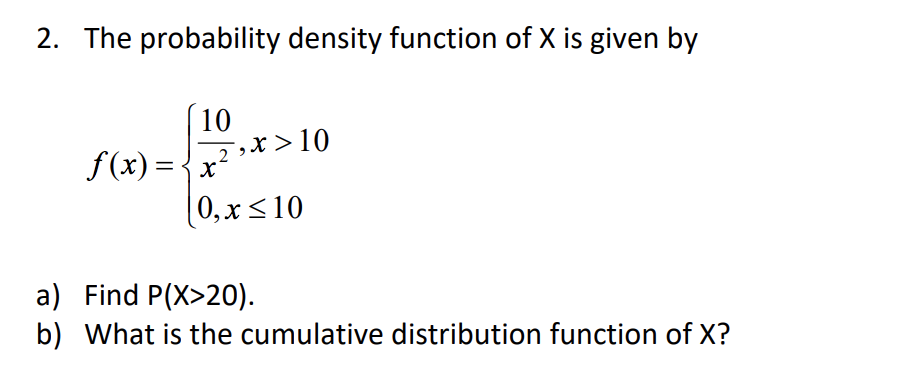2. The probability density function of X is given by 10 0,x < 10 a) Find P(X>20). b) What is the cumulative distribution function of X?

• ### (1 point) 3. Let X and Y be random variables with a joint probability density function...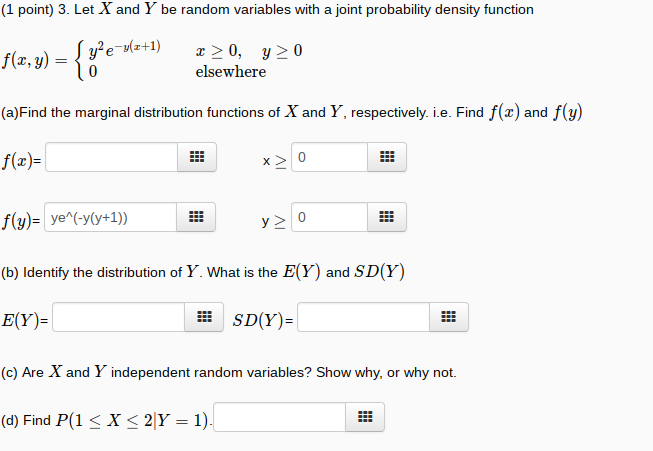(1 point) 3. Let X and Y be random variables with a joint probability density function f(z, y)e (a)Find the marginal distribution functions of X and Y, respectively. i.e. Find f(z) and f(y) f(x)- elsewhere (b) Identify the distribution of Y. What is the E(Y) and SD(Y) E(Y)- (c) Are X and Y independent random variables? Show why, or why not (d) Find P(1 X 2|Y 1) E SD(Y)-

• ### 1. 20 points Let X be a random variable with the following probability density function: f(x)--e+1"...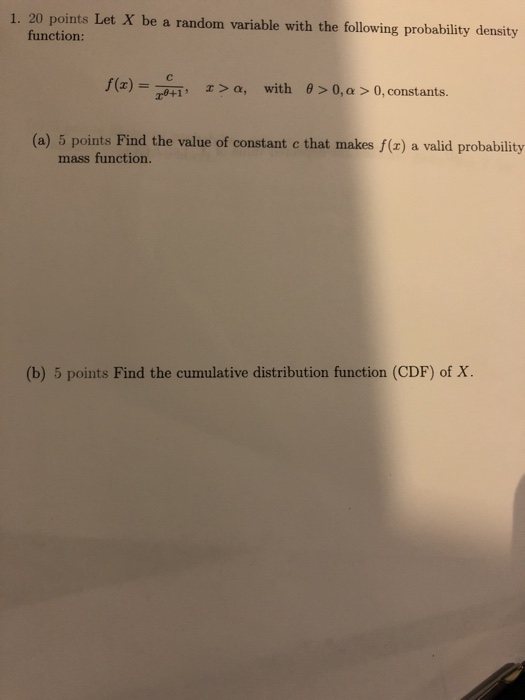1. 20 points Let X be a random variable with the following probability density function: f(x)--e+1" with ? > 0, ? > 0, constants x > ?, (a) 5 points Find the value of constant c that makes f(x) a valid probability mass function. (b) 5 points Find the cumulative distribution function (CDF) of X.

• ### Let X have probability density function f(2)= k(1+x) -3 for 0 < x < oo and...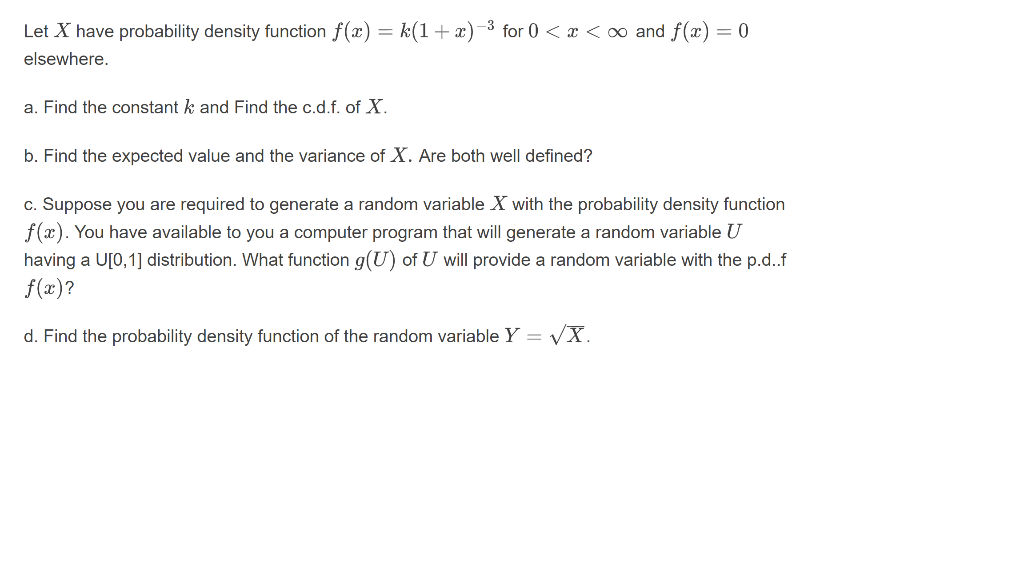Let X have probability density function f(2)= k(1+x) -3 for 0 < x < oo and f(x) = 0 elsewhere. a. Find the constant k and Find the c.d.f. of X. b. Find the expected value and the variance of X. Are both well defined? c. Suppose you are required to generate a random variable X with the probability density function f(x). You have available to you a computer program that will generate a random variable U having a U[0,...

• ### Assume that the joint density function of X and Y is given by f (x, y)...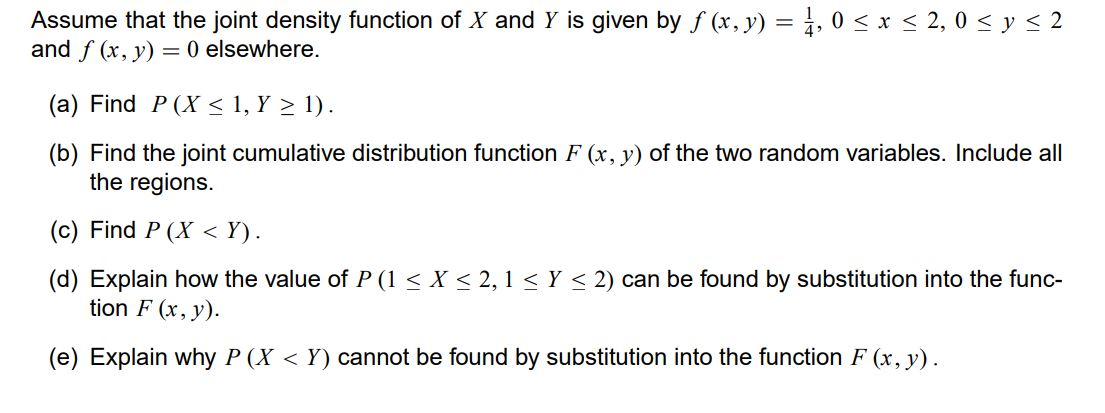Assume that the joint density function of X and Y is given by f (x, y) = 4,0 < x < 2,0 < y = 2 and f (x, y) = 0 elsewhere. (a) Find P (X < 1, Y > 1). (b) Find the joint cumulative distribution function F(x, y) of the two random variables. Include all the regions. (c) Find P (X<Y). (d) Explain how the value of P (1 < X < 2,1 < Y < 2)...

• ### Find the mean of X given Y = 1/2. The joint probability density function is f(x,...

Find the mean of X given Y = 1/2. The joint probability density function is f(x, y) for random variables X and Y. f(x, y) = { (12/7)(xy + y^2) 0 < x < 1, 0 < y < 1 0 elsewhere

• ### How to get the cdf when y>x>0? Thanks 6. The joint probability density function (pdf) of...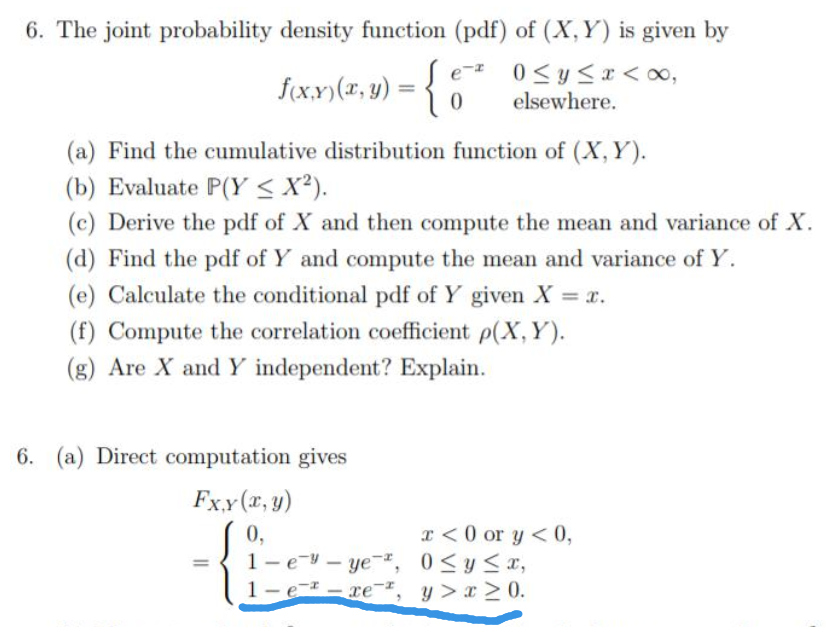How to get the cdf when y>x>0? Thanks 6. The joint probability density function (pdf) of (X, Y) is given by 0y<oo, elsewhere. fxr, y) (a) Find the cumulative distribution function of (X, Y) (b) Evaluate P(Y < X2) (c) Derive the pdf of X and then compute the mean and variance of X (d) Find the pdf of Y and compute the mean and variance of Y (e) Calculate the conditional pdf of Y given X (f) Compute the...